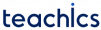# Flow Meters

Flow meters are the devices used for measuring the flow rate of liquids or gases. Flow rate can be defined as the volume of fluid flowing through an area in unit time. In a pipe, the flow rate can be expressed in terms of speed of the fluid and the cross-sectional area of the pipe.

## Obstruction Type Flow meter

Different types of flow measuring techniques are used based on the requirements and depending upon the situation. Common types of flow meters include

In an obstruction type flow meter, an obstruction is created in the flow passage and the pressure drop across the obstruction is measured. This change in pressure is calibrated in terms of flow rate.

Depending upon the type of obstruction, different types of obstruction flow meters such as Orifice meter, Venturimeter, Flow nozzle, etc. are available.

### Orifice meter

In an orifice type flow meter, an orifice plate is placed in the pipe as shown in the figure. The pressure is dropped suddenly when the fluid passes the orifice and continues to drop until ‘vena contracta‘ is reached. It is a result of the increase in velocity of the fluid passing through the reduced area.

Vena contracta is the point in a fluid stream where the diameter of the stream is the least.

After vena contracta, the pressure increases gradually until a maximum pressure point is reached that will be lower than the pressure P1. The flow rate can be obtained by measuring the pressure difference (P1-P2).

The major advantages of the orifice plate that it is simple in construction, low-cost device and easy to install. The main disadvantage of using the orifice plate is the permanent pressure drop occurred in the orifice plate. This problem can be overcome by using Venturimeter or Flow nozzles.

### Venturimeter

A venturimeter is constructed in such a way that change in the flow path is gradual. As a result, no permanent pressure drop has occurred in the flow path.

The middle portion of the venturimeter is called the throat. The crossectional area of this area is too small. Here pressure decreases and velocity increases.

The starting section of venturimeter where the crossectional area starts to decrease is called converging section. The end of the converging section is attached with the throat.

The last part of the venturimeter is called the diverging part. The crossectional area of this section increases continually.

Venturimeter works on Bernoulli’ equation. That is pressure decreases with increasing velocity. Since the crossectional area of the throat section is smaller, the velocity of flow will be higher in this area. This happens according to the continuity equation. This increase in velocity results in a decrease in pressure. This change in pressure is measured to calculate the flow rate of the fluid.

The main disadvantage of venturimeter is that the cost of making is high.

### Flow nozzle

Flow nozzles are a compromise between the orifice plate and venturimeter both in cost and performance. Typical construction of a flow nozzle is shown in the figure.

The shape of the flow nozzle provides lesser resistance to flow when compared to an orifice meter. It has no divergent cone for pressure recovery as in a venturimeter.

Flow nozzles are compact, cheaper and easy install as compared to venturimeter. Since there is no divergent cone pressure recovery is low when compared to venturimeter.

### Rotameter

The orifice meter, Venturimeter and flow nozzle work on the principle of constant area variable pressure drop. Here the pressure drop changes with flow rate and the area of obstruction are always constant. The rotameter works as a constant pressure drop variable area meter. That is the pressure drop is always constant and the area of obstruction is changed.

Typical construction of a rotameter is shown in the figure. It consists of a tapered pipe and a floating element. The float has specific gravity higher than the fluid. When the fluid starts to flow through the pipe, the float rises due to the buoyancy, increasing the area for passage of the fluid. The movement of the float will directly proportional to the flow rate of the fluid. Flow rate can be measured when the float reaches a stable position where the force exerted by the fluid equals the gravitational force exerted by the float.

Rotometers are simple to operate, easy to handle and the cost of making is low. It doesn’t require external power or energy for its operation.

Subscribe
Notify of